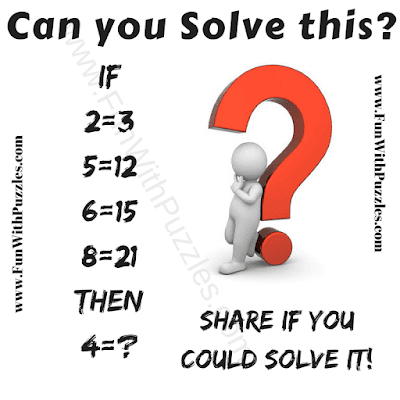Here is the Maths Logical Question in which there are some logical equations. Your challenge is to decode the logical patterns in these equations and solve the last equation to find the value of the missing number which will replace the question mark?Can you solve this Maths Logical Question?

The answer to this "Maths Logical Question", can be viewed by clicking on the button. Please do give your best try before looking at the answer.

#### 1 comment:

GRLTejaswi said...

9